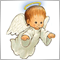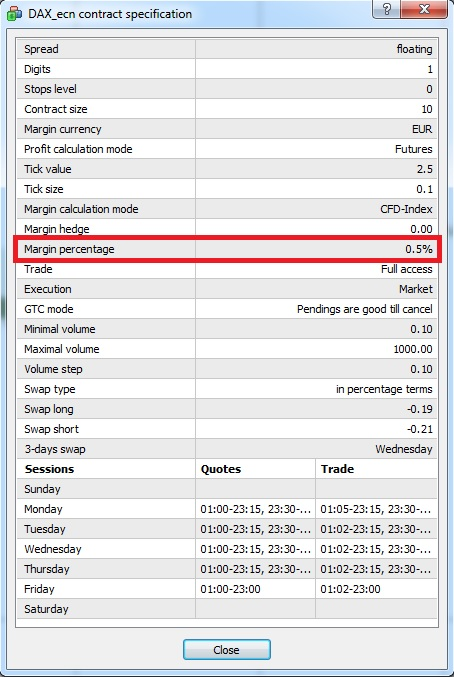# Leverage for each symbol3531

I know it isn't a new problem but with new ESMA rules (about leverages) it became "interesting" for me. Generally now we have a different leverage for each symbol. But I know only the function AccountLeverage() (or with the same result AccountInfoInteger(ACCOUNT_LEVERAGE) ) that provides something like an account leverage but it is useless now. I am convinced that MQL4 (and I believe MQL5 too) doesn't have such a function that provides a leverage for a specific symbol. But maybe I'm wrong. Then my question is: Is there such a function in MQL4? Or we have to calculate the leverage for a specific symbol by ourselves (e.g. by AccountFreeMarginCheck)?

It would be nice if someone has already written such a function and would like to share it.79

Petr Nosek:

Hi Petr,

Is this what you are looking for?

Swap.mq4: this is a script, so you should put it in your scripts folder. Drag it over the chart of a specific pair and it will show you the margin, swaps and some other info for this specific pair.

PositionSizeCalculator.mq4 is an indicator, so you should put it in your indicators folder. Drag it over the chart of a specific pair, click OK on the pop-up window (tried to get rid of it but could not do it... If you can do this, please let me know) and it will show you the margin and some other info for this specific pair.

I am not the author of both of these files, I just simplified their code to make them easier for my use...

Files:
Swaps.mq4 8 kb3531

rosspet6:

Hi Petr,

Is this what you are looking for?

Swap.mq4: this is a script, so you should put it in your scripts folder. Drag it over the chart of a specific pair and it will show you the margin, swaps and some other info for this specific pair.

PositionSizeCalculator.mq4 is an indicator, so you should put it in your indicators folder. Drag it over the chart of a specific pair, click OK on the pop-up window (tried to get rid of it but could not do it... If you can do this, please let me know) and it will show you the margin and some other info for this specific pair.

I am not the author of both of these files, I just simplified their code to make them easier for my use...

Hi rosspet6,
Thank you for your effort but I don't need scripts or indicators that write out info about the symbol or calculate position (I have my own). I was just curious if I didn't overlook an MQL function that provides a leverage for the specific symbol. It seems to me that the function doesn't exist in MQL and I'll have to code my own.3531

It seems that nobody wants to share his/her code that provides leverage for each symbol. I started researching how to calculate the leverage by available MQL4 functions. Till now I've found out that there are two different way how to calculate a leverage. It depends on the value MarketInfo(symbol,MODE_MARGINCALCMODE). We have to calculate the leverage in a different way for Forex (the value is equal to zero) and in different way for other values of MARGINCALCMODE (CFD, CFD-Indexes, CFD -Leverage... in other words if the value is greater than zero). Both of them are really simply formulas. Maybe we have to use another formula for some specific value of MARGINCALCMODE (I've tested at my brokers and I guess we don't need another formula).

BUT unfortunately I've encountered with my old problem (see https://www.mql5.com/en/forum/227407) about tick value. Some brokers provide tick value for some symbols in base currency. MQL4 documentation says that the tick value should be in account (deposit) currency. And this causes problem because there isn't a reliable way how to find out in what currency the tick value is (IMHO).

Maybe there is a way to confirm the leverage calculation (and then also an used tick value currency). I've encountered with a property in the window "Symbol specification" called "Margin percentage" (see attached picture). I think I would be able to calculate a leverage by using this value. But I don't know how to get this value by MQL4 function. If somebody knows let me know. Thank in advance.40335

Petr Nosek:

It seems that nobody wants to share his/her code that provides leverage for each symbol. I started researching how to calculate the leverage by available MQL4 functions. Till now I've found out that there are two different way how to calculate a leverage. It depends on the value MarketInfo(symbol,MODE_MARGINCALCMODE). We have to calculate the leverage in a different way for Forex (the value is equal to zero) and in different way for other values of MARGINCALCMODE (CFD, CFD-Indexes, CFD -Leverage... in other words if the value is greater than zero). Both of them are really simply formulas. Maybe we have to use another formula for some specific value of MARGINCALCMODE (I've tested at my brokers and I guess we don't need another formula).

BUT unfortunately I've encountered with my old problem (see https://www.mql5.com/en/forum/227407) about tick value. Some brokers provide tick value for some symbols in base currency. MQL4 documentation says that the tick value should be in account (deposit) currency. And this causes problem because there isn't a reliable way how to find out in what currency the tick value is (IMHO).

Maybe there is a way to confirm the leverage calculation (and then also an used tick value currency). I've encountered with a property in the window "Symbol specification" called "Margin percentage" (see attached picture). I think I would be able to calculate a leverage by using this value. But I don't know how to get this value by MQL4 function. If somebody knows let me know. Thank in advance.

This value is what you are calculating, there is no mql4 function giving it. Your leverage is 200, not ?3531

Alain Verleyen:
This value is what you are calculating, there is no mql4 function giving it. Your leverage is 200, not ?
I thought it that there isn't such a function giving it. But it is interesting that this value is always right even if the tick value is in base currency. It must be either calculate in different way from my way or stored and unavailable for MQL4.
BTW the leverage for the example from my picture is 20.40335

Petr Nosek:
I thought it that there isn't such a function giving it. But it is interesting that this value is always right even if the tick value is in base currency. It must be either calculate in different way from my way or stored and unavailable for MQL4.
BTW the leverage for the example from my picture is 20.

How is that "this value is always right" if the leverage is 20 ?

Which broker is it ?3531

Alain Verleyen:

How is that "this value is always right" if the leverage is 20 ?

Which broker is it ?

I've tested this relations at four brokers.40335

Petr Nosek:
I've tested this relations at four brokers.

I tested one with leverage 20, and the "Margin percentage" value was 5%.3531

Leverage relations among:

1. Margin calc mode
2. Margin percentage
3. Contract size

by my research are:

Margin calc mode
Leverage
0 - Forex
100 / MarginPercentage
1 - CFD
1 / MarginPercentage
2 - ???    My brokers don't have such a symbol with 2 as Margin calc mode
???
3 - CFD-Index
1 / (MarginPercentage * ContractSize)
4 - CFD-Leverage  100 / MarginPercentage

But unfortunately we can't get the value of Margin Percentage by MQL4 function.3531

Alain Verleyen: